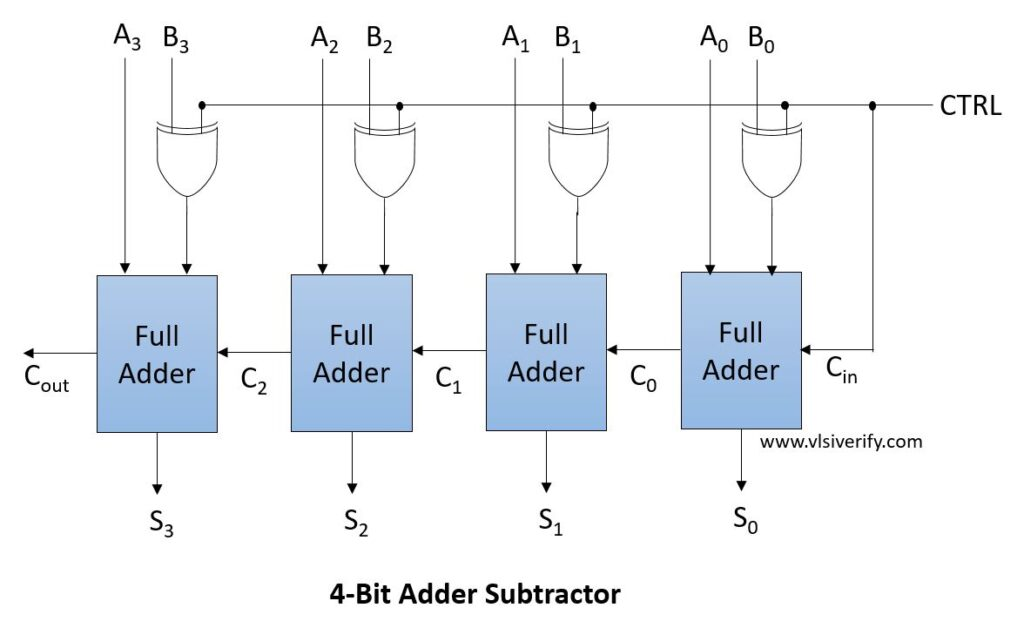4-bit Adder Subtractor - VLSI Verify

Tutorials

When control bit CTRL decides whether to do addition or subtraction for two 4 bit integers A and B. The signal CTRL is attached to one of the inputs of the XOR gate and another input is connected to B.

CTRL = 0: Addition is performed (A + B)

CTRL = 1: Subtraction is performed. XOR gate output becomes a complement of B. So, addition is performed as A + (-B)

If Cout = 1, ignore it and sum S is the answer and if it is 0, Sum S represents 2’s complement of the answer and the number is negative.

## Block Diagram## 4 bit Adder Subtractor Verilog Code

``````module full_adder(
input a, b, cin,
output sum, cout
);

assign {sum, cout} = {a^b^cin, ((a & b) | (b & cin) | (a & cin))};
//or
//assign sum = a^b^cin;
//assign cout = (a & b) | (b & cin) | (a & cin);
endmodule

module ripple_carry_adder_subtractor #(parameter SIZE = 4) (
input [SIZE-1:0] A, B,
input CTRL,
output [SIZE-1:0] S, Cout);
bit [SIZE-1:0] Bc;
genvar g;

assign Bc = B ^ CTRL;

full_adder fa0(A, Bc, CTRL, S, Cout);
generate  // This will instantial full_adder SIZE-1 times
for(g = 1; g<SIZE; g++) begin
assign Bc[g] = B[g] ^ CTRL;
full_adder fa(A[g], Bc[g], Cout[g-1], S[g], Cout[g]);
end
endgenerate
endmodule
``````

### Testbench Code

``````module RCAS_TB;
wire [3:0] S, Cout;
reg [3:0] A, B;
reg ctrl;

ripple_carry_adder_subtractor rcas(A, B, ctrl, S, Cout);

initial begin
\$monitor("CTRL=%b: A = %b, B = %b --> S = %b, Cout = %b", ctrl, A, B, S, Cout);
ctrl = 0;
A = 1; B = 0;
#3 A = 2; B = 4;
#3 A = 4'hb; B = 4'h6;
#3 A = 5; B = 3;
ctrl = 1;
A = 1; B = 0;
#3 A = 2; B = 4;
#3 A = 4'hb; B = 4'h6;
#3 A = 5; B = 3;
#3 \$finish;
end

initial begin
\$dumpfile("waves.vcd");
\$dumpvars;
end
endmodule
``````

Output:

``````CTRL=0: A = 0001, B = 0000 --> S = 0001, Cout = 0
CTRL=0: A = 0010, B = 0100 --> S = 0110, Cout = 0
CTRL=0: A = 1011, B = 0110 --> S = 0001, Cout = 1
CTRL=1: A = 0001, B = 0000 --> S = 0001, Cout = 1
CTRL=1: A = 0010, B = 0100 --> S = 1110, Cout = 0
CTRL=1: A = 1011, B = 0110 --> S = 0101, Cout = 1
CTRL=1: A = 0101, B = 0011 --> S = 0010, Cout = 1``````

Verilog Codes